# Bose-Einstein statistics

Also found in: Dictionary, Thesaurus, Acronyms.
Related to Bose-Einstein statistics: Fermi-Dirac statistics, Bose-Einstein condensate

## Bose-Einstein statistics,

class of statistics that applies to elementary particles called bosons, which include the photonphoton
, the particle composing light and other forms of electromagnetic radiation, sometimes called light quantum. The photon has no charge and no mass. About the beginning of the 20th cent.
, pionpion
or pi meson,
lightest of the meson family of elementary particles. The existence of the pion was predicted in 1935 by Hideki Yukawa, who theorized that it was responsible for the force of the strong interactions holding the atomic nucleus together.
, and the W and Z particlesW and Z particles,
elementary particles that mediate, or carry, the fundamental force associated with weak interactions. The discovery of the W and Z particles at CERN near Geneva, Switzerland, in the early 1980s was an important confirmation of electroweak theory,
. Bosons have integral values of the quantum mechanical property called spin and are "gregarious" in the sense that an unlimited number of bosons can be placed in the same state. All of the particles that mediate the fundamental forces of nature are bosons. See elementary particleselementary particles,
the most basic physical constituents of the universe. Basic Constituents of Matter

Molecules are built up from the atom, which is the basic unit of any chemical element. The atom in turn is made from the proton, neutron, and electron.
; Fermi-Dirac statisticsFermi-Dirac statistics,
class of statistics that applies to particles called fermions. Fermions have half-integral values of the quantum mechanical property called spin and are "antisocial" in the sense that two fermions cannot exist in the same state.
; statistical mechanicsstatistical mechanics,
quantitative study of systems consisting of a large number of interacting elements, such as the atoms or molecules of a solid, liquid, or gas, or the individual quanta of light (see photon) making up electromagnetic radiation.
.

## Bose-Einstein statistics

The statistical description of quantum mechanical systems in which there is no restriction on the way in which particles can be distributed over the individual energy levels. This description applies when the system has a symmetric wave function. This in turn has to be the case when the particles described are of integer spin.

Suppose one describes a system by giving the number of particles ni in an energy state εi, where the ni are called occupation numbers and the index i labels the various states. The energy level εi, is of finite width, being really a range of energies comprising, say, gi, individual (nondegenerate) quantum levels. If any arrangement of particles over individual energy levels is allowed, one obtains for the probability of a specific distribution Eq. (1a). In Boltzmann statistics, this same probability would be written as Eq.

(1 a)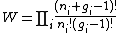(1 b)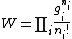(1b). See Boltzmann statistics

The equilibrium state is defined as the most probable state of the system. To obtain it, one must maximize Eq. (1a) under the conditions given by Eqs. (2a)

(2 a)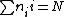(2 b)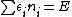and (2b), which express the fact that the total number of particles N and the total energy E are fixed. One finds for the most probable distribution that Eq. (3) holds.
(3)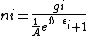Here, A and ß are parameters to be determined from Eqs. (2a) and (2b); actually, ß\,\, = 1/kT, where k is the Boltzmann constant and T is the absolute temperature.

An interesting and important result emerges when Eq. (3) is applied to a gas of photons, that is, a large number of photons in an enclosure. (Since photons have integer spin, this is legitimate.) The formula for the energy density (energy per unit volume) in a given frequency range is found to be the celebrated Planck radiation formula for blackbody radiation. Thus, black-body radiation must be considered as a photon gas, with the photons satisfying Bose-Einstein statistics. See Heat radiation

If an ideal Bose gas, consisting of a fixed number of material particles, is compressed beyond a certain point, some of the particles will condense in a zero state, where they do not contribute to the density or the pressure. If the volume is decreased, this curious condensation phenomenon results, yielding the zero state which has the paradoxical properties of not contributing to the pressure, volume, or density. There is now considerable evidence that many of the superfluid properties exhibited by liquid helium are in fact manifestations of an Einstein condensation. See Fermi-Dirac statistics, Quantum statistics, Statistical mechanics

McGraw-Hill Concise Encyclopedia of Physics. © 2002 by The McGraw-Hill Companies, Inc.
The following article is from The Great Soviet Encyclopedia (1979). It might be outdated or ideologically biased.

## Bose-Einstein Statistics

physical quantum statistics; applicable to systems of particles with zero or integral spin. It was proposed in 1924 by S. Bose for light quanta and developed (in the same year) by A. Einstein as applied to the study of the molecules of ideal gases. The characteristic property of Bose-Einstein statistics consists in the fact that any number of identical particles can exist in the same state (in contrast with Fermi-Dirac statistics for particles with half-integral spin, according to which each state can be occupied by not more than one particle). For highly rarefied gases and high temperatures, Bose-Einstein statistics (like Fermi-Dirac statistics) becomes Boltzmann statistics.